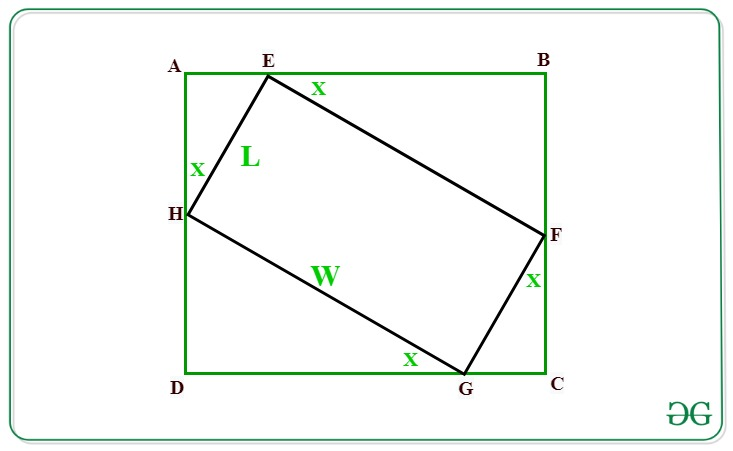# Maximum area of a Rectangle that can be circumscribed about a given Rectangle of size LxW

• Last Updated : 27 Apr, 2021

Given a rectangle of dimensions L and W. The task is to find the maximum area of a rectangle that can be circumscribed about a given rectangle with dimensions L and W.

Examples:

Input: L = 10, W = 10
Output: 200

Input: L = 18, W = 12
Output: 450

Approach: Let below is the given rectangle EFGH of dimensions L and W. We have to find the area of rectangle ABCD which is circumscribing rectangle EFGHIn the above figure:
Ifthenas GCF is right angled triangle.
Therefore,=>=>Similarly,Now, The area of rectangle ABCD is given by:

Area = AB * AD
Area = (AE + EB)*(AH + HD) …..(1)

According to the projection rule:
AE = L*sin(X)
EB = W*cos(X)
AH = L*cos(X)
HD = W*sin(X)

Substituting the value of the above projections in equation (1) we have:Now to maximize the area, the value of sin(2X) must be maximum i.e., 1.
Therefore after substituting sin(2X) as 1 we have,Below is the implementation of the above approach:

## C++

 // C++ program for the above approach#include using namespace std; // Function to find area of rectangle// inscribed another rectangle of// length L and width Wdouble AreaofRectangle(int L, int W){         // Area of rectangle    double area = (W + L) * (W + L) / 2;         // Return the area    return area;} // Driver Codeint main(){         // Given dimensions    int L = 18;    int W = 12;         // Function call    cout << AreaofRectangle(L, W);    return 0;} // This code is contributed by Princi Singh

## Java

 // Java program for the above approachimport java.io.*;import java.util.*; class GFG{     // Function to find area of rectangle// inscribed another rectangle of// length L and width Wstatic double AreaofRectangle(int L, int W){         // Area of rectangle    double area = (W + L) * (W + L) / 2;         // Return the area    return area;}     // Driver Codepublic static void main(String args[]){         // Given dimensions    int L = 18;    int W = 12;         // Function call    System.out.println(AreaofRectangle(L, W));}} // This code is contributed by offbeat

## Python3

 # Python3 program for the above approach # Function to find area of rectangle# inscribed another rectangle of# length L and width Wdef AreaofRectangle(L, W):     # Area of rectangle  area =(W + L)*(W + L)/2 # Return the area  return area # Driver Codeif __name__ == "__main__":   # Given Dimensions  L = 18  W = 12   # Function Call  print(AreaofRectangle(L, W))

## C#

 // C# program for the above approachusing System; class GFG{     // Function to find area of rectangle// inscribed another rectangle of// length L and width Wstatic double AreaofRectangle(int L, int W){         // Area of rectangle    double area = (W + L) * (W + L) / 2;         // Return the area    return area;}     // Driver Codepublic static void Main(String []args){         // Given dimensions    int L = 18;    int W = 12;         // Function call    Console.Write(AreaofRectangle(L, W));}} // This code is contributed by shivanisinghss2110

## Javascript

 

Output:

450.0

Time Complexity: O(1)
Auxiliary Space: O(1)

My Personal Notes arrow_drop_up